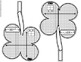# Saint Patrick's Day Algebra Graphing Exponential Functions Shamrock Math PennantSubject
Resource Type
File Type

PDF

(3 MB|9 pages)
Standards
Also included in:
1. This bundle currently includes 38 algebra pennants and will be updated with additional sets when they are created. You will be notified when a new set is added and will be able to re-download to get the new sets free of charge. Math pennants are a fun way to show off student work, create colorful bu
\$123.00
\$62.50
Save \$60.50
• Product Description
• StandardsNEW

Students graph exponential functions in this math pennant activity that doubles as student-created classroom decor. Each pennant is shaped like a shamrock to celebrate St. Patrick's Day in your algebra classroom.

*This is the same activity as Valentine's Day Algebra Graphing Exponential Functions Hearts Math Pennant, only with a St. Patrick's Day theme.

All exponential functions are growth functions and the problems vary in difficulty, from functions with only vertical shifts to functions with vertical and horizontal shifts. Each pennant includes a table for students to fill in to find points to plot. The coordinates in the answer key are based on coordinates found after shifting parent graphs. You can find a free graphing exponential functions cheat sheet to go along with this activity here:

This activity includes 20 shamrock pennants that vary in difficulty and an answer key with graphs and completed tables.

You may also like:

Algebra Activities Bundle

Use the properties of exponents to interpret expressions for exponential functions. For example, identify percent rate of change in functions such as y = (1.02) to the 𝘵 power, 𝘺 = (0.97) to the 𝘵 power, 𝘺 = (1.01) to the 12𝘵 power, 𝘺 = (1.2) to the 𝘵/10 power, and classify them as representing exponential growth or decay.
Graph exponential and logarithmic functions, showing intercepts and end behavior, and trigonometric functions, showing period, midline, and amplitude.
Graph functions expressed symbolically and show key features of the graph, by hand in simple cases and using technology for more complicated cases.
Explain why the 𝘹-coordinates of the points where the graphs of the equations 𝘺 = 𝘧(𝘹) and 𝘺 = 𝑔(𝘹) intersect are the solutions of the equation 𝘧(𝘹) = 𝑔(𝘹); find the solutions approximately, e.g., using technology to graph the functions, make tables of values, or find successive approximations. Include cases where 𝘧(𝘹) and/or 𝑔(𝘹) are linear, polynomial, rational, absolute value, exponential, and logarithmic functions.
Total Pages
9 pages
Included
Teaching Duration
N/A
Report this Resource to TpT
Reported resources will be reviewed by our team. Report this resource to let us know if this resource violates TpT’s content guidelines.